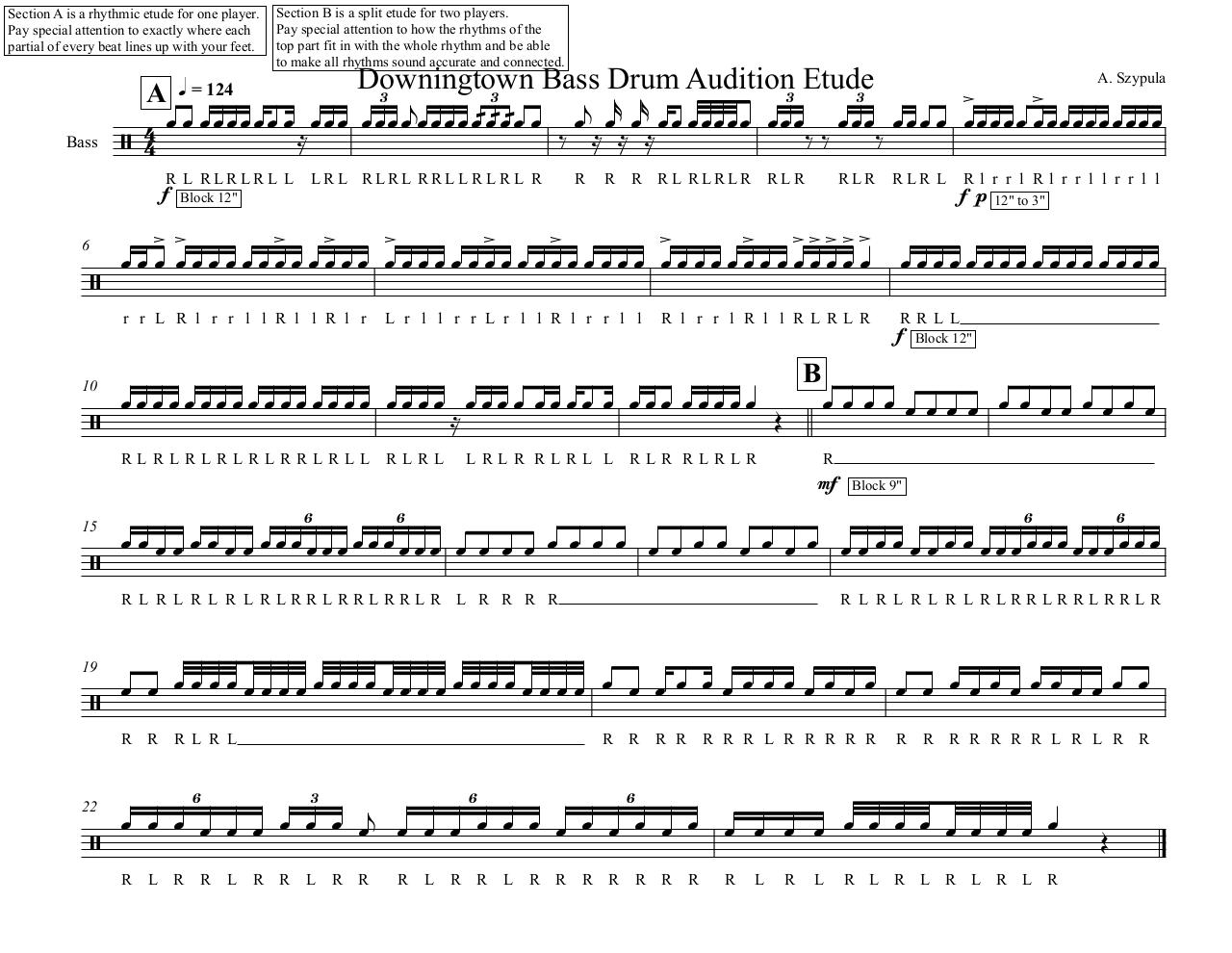Downingtown Bass Drum Audition .pdf

File information

Original filename: Downingtown Bass Drum Audition.pdf
Title: Downingtown Bass Drum Audi
Author: Andrew Szypula

This PDF 1.3 document has been generated by Sibelius 5 / Mac OS X 10.7.2 Quartz PDFContext, and has been sent on pdf-archive.com on 04/12/2011 at 00:20, from IP address 144.26.x.x. The current document download page has been viewed 1234 times.
File size: 69 KB (1 page).
Privacy: public file

Downingtown Bass Drum Audition.pdf (PDF, 69 KB)

Document preview

Section A is a rhythmic etude for one player.
Pay special attention to exactly where each
partial of every beat lines up with your feet.

Bass

Section B is a split etude for two players.
Pay special attention to how the rhythms of the
top part fit in with the whole rhythm and be able
to make all rhythms sound accurate and connected.

Downingtown
Bass Drum Audition3 Etude
A q = 124
3
3
3

          











   


R L RLRLRL L LRL RLRL RRL LR L R L R
f Block 12"

6

10

A. Szypula

R

R R RL RLRLR

RLR

RLR


 

 
   
r r L R l r r l l R l l R l r

L r l l r r L r l l R l r r l l

R l r r l R l l RL RL R

B

      

RLRLRLRLRLRRLRLL RLRL

LRLR RLRL L RLR RLRLR

RLR L

Rl r r l Rl r r l l r r l l
f p 12" to 3"


RRL L
f Block 12"

 
R

mf Block 9"
15

19

22

6

6

6

6

                                 
R L R L R L R L RLRRLRRLRRLR L R R R R

R L R L R L R L RLRRLRRLRRLR

                           
R R R L R L

R R R R R R R L R R R R R

R R R R R R R L R L R R

                                  
6

R

L R R

3

L R R

L R R

6

R

L R R

6

L R R R R R R R

R

L

R

L

R L R L R L R L RHTML Code

Copy the following HTML code to share your document on a Website or Blog

QR CodeRelated keywords